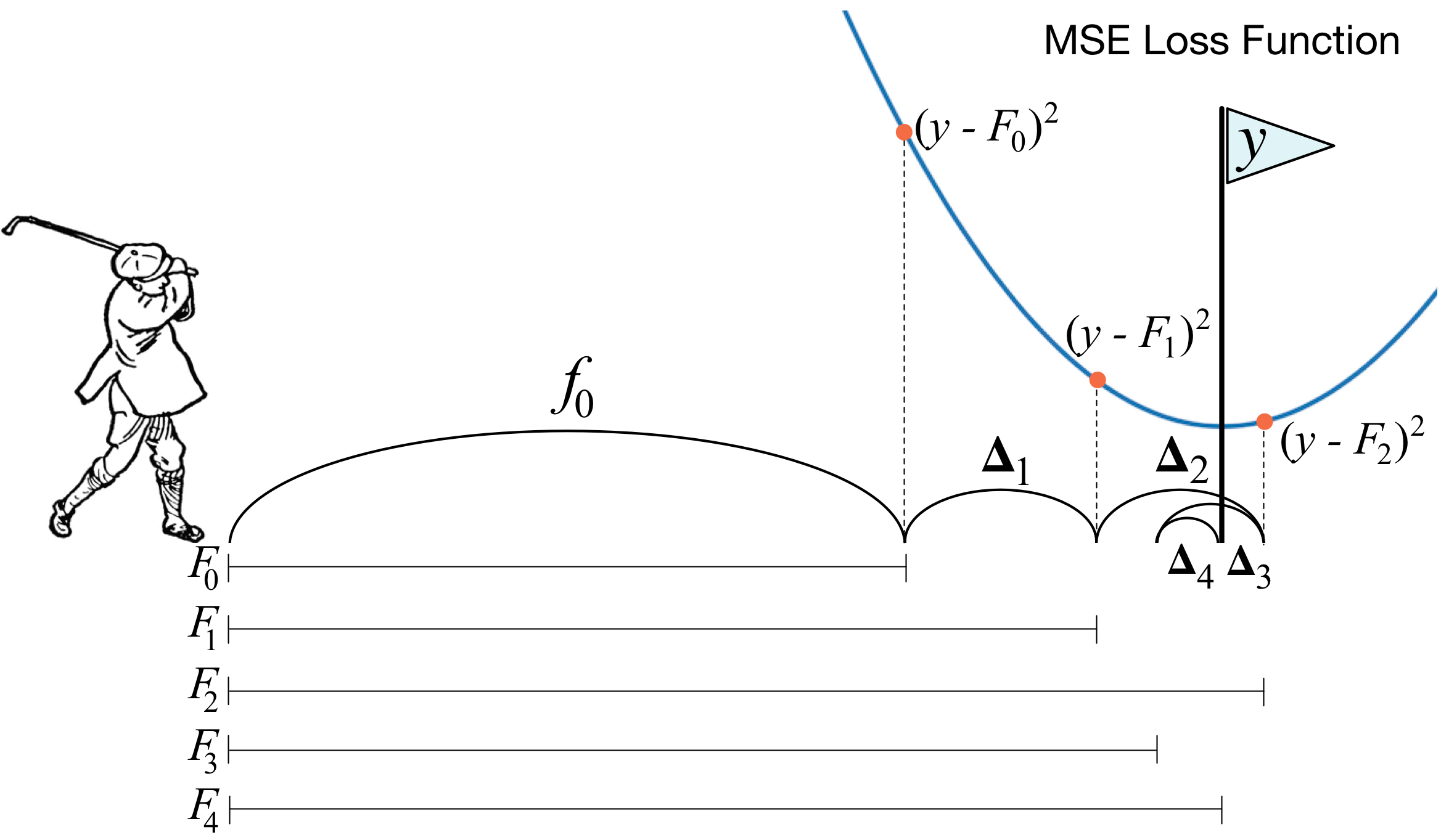# How to explain gradient boosting

Brought to you by explained.ai

(We teach in University of San Francisco's MS in Data Science program and have other nefarious projects underway. You might know Terence as the creator of the ANTLR parser generator. Jeremy is a founding researcher at fast.ai, a research institute dedicated to making deep learning more accessible.)Gradient boosting machines (GBMs) are currently very popular and so it's a good idea for machine learning practitioners to understand how GBMs work. The problem is that understanding all of the mathematical machinery is tricky and, unfortunately, these details are needed to tune the hyper-parameters. (Tuning the hyper-parameters is required to get a decent GBM model unlike, say, Random Forests.) Our goal in this article is to explain the intuition behind gradient boosting, provide visualizations for model construction, explain the mathematics as simply as possible, and answer thorny questions such as why GBM is performing “gradient descent in function space.” We've split the discussion into three morsels and a FAQ for easier digestion.

1. Gradient boosting: Distance to target.
First, we examine the most common form of GBM that optimizes the mean squared error (MSE), also called the L2 loss or cost. (The mean squared error is the average of the square of the difference between the true targets and the predicted values from a set of observations, such as a training or validation set.) As we'll see, A GBM is a composite model that combines the efforts of multiple weak models to create a strong model, and each additional weak model reduces the mean squared error (MSE) of the overall model. We give a fully-worked GBM example for a simple data set, complete with computations and model visualizations.

Optimizing a model according to MSE makes it chase outliers because squaring the difference between targets and predicted values emphasizes extreme values. When we can't remove outliers, it's better to optimize the mean absolute error (MAE), also called the L1 loss or cost. (The MAE is the average of the absolute value of the difference between the true targets and the predicted values.) This second article shows the computations and visualizations for a GBM that optimizes MAE for the same data set as used in the first article to optimize MSE.

The previous two articles give the intuition behind GBM and the simple formulas to show how weak models join forces to create a strong regression model. No attempt was made to show how we can abstract out a generalized GBM that works for any loss function. This last article demonstrates that gradient boosting is really doing a form of gradient descent and, therefore, is in fact optimizing MSE or MAE depending on the direction vectors we use to train the weak models. The discussion relies on a bit of derivative calculus but it's an important read if you'd like to learn deeply how GBM works. (To brush up on your vectors and derivatives, you can check out The Matrix Calculus You Need For Deep Learning.) We finish off by clearing up a number of confusion points regarding gradient boosting.

As Ben Gorman points out in A Kaggle Master Explains Gradient Boosting, “This is the part that gets butchered by a lot of gradient boosting explanations.” His blog post does a good job of explaining it, but we give our own perspective here.